After a concise investigation on the P2’s oscillator and slew rate response, we proceed with the diode bridge and offset adjust blocks. A diode bridge can be configured as a double balanced modulator/mixer by adding transformers that “multiply” the signals, forming 2 sidebands. Remember that we can represent the trigonometric functions sin(x) or cos(x) with their complex exponential counterparts. When we do this for the product of 2 trigonometric functions and simplify, we end up with the sum of 2 trigonometric functions whose frequencies have been added and subtracted to each other. Therefore, we expect the output of the diode bridge to be a resultant wave composed of f1+f2 and f1-f2, given that f1 and f2 are the frequencies of the 2 input signals to the bridge.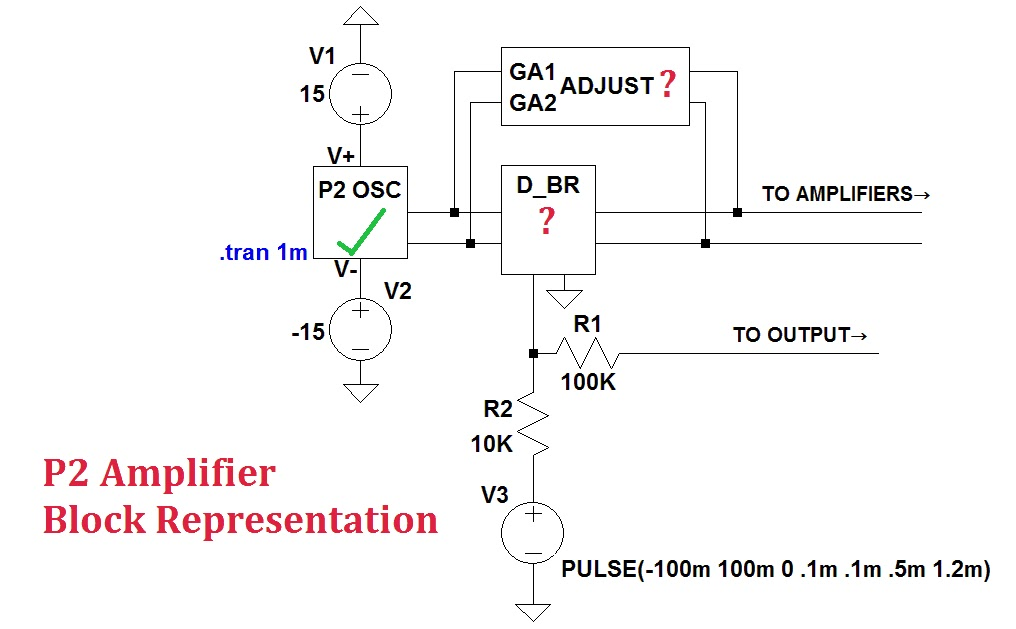Figure 1. Simplified block representation showing how the oscillator, diode bridge, P2 inputs and gain/phase adjust interact.

By doing this, DC-related noise is significantly reduced (since information from the input signal is now “encoded” in AC).

To get a better feel of the diode bridge, I have prepared a test setup below.Figure 2. The internal schematic of the P2 diode bridge.
The inductors provide DC isolation, with a coupling coefficient of 1. Diodes D1, D2, D3, and D4 are identical, modelled with a zero-bias junction capacitance of 105pF [default=0] and saturation current of 3pF [default=0.01 pA]. The signal entering OPAMPIN+ to OPAMPIN- will be superimposed on the signal entering OSCIN1 to OSCIN2. The output is at DBROUT1, with its 180° phase shifted version at DBROUT2.

### Coupling 5MHz and 1 kHz sinusoids with the Diode Bridge.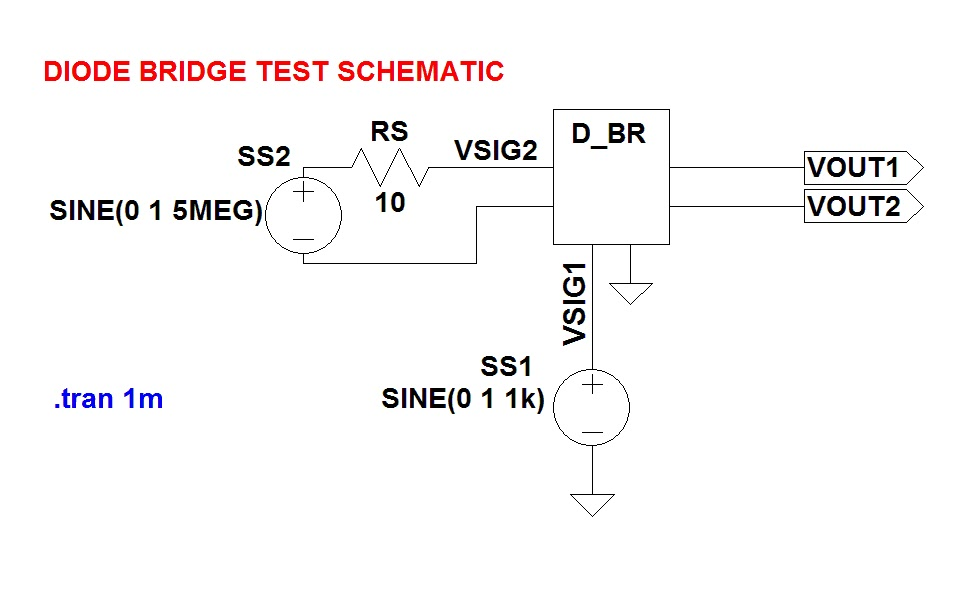Figure 3. Diode bridge test schematic for Case 1.

First, I test what will happen when I feed a 1 kHz and a 5 MHz sinusoid to the diode bridge block of the P2. Signal source/generator SS1 will supply the 1V pk-pk 1kHz sine wave while SS2 will provide a 5MHz signal of equal amplitude. I am theoretically expecting a signal with pronounced frequency components at 5MHz+1kHz and 5MHz-1kHz. Simulating yields the VOUT1 waveform below.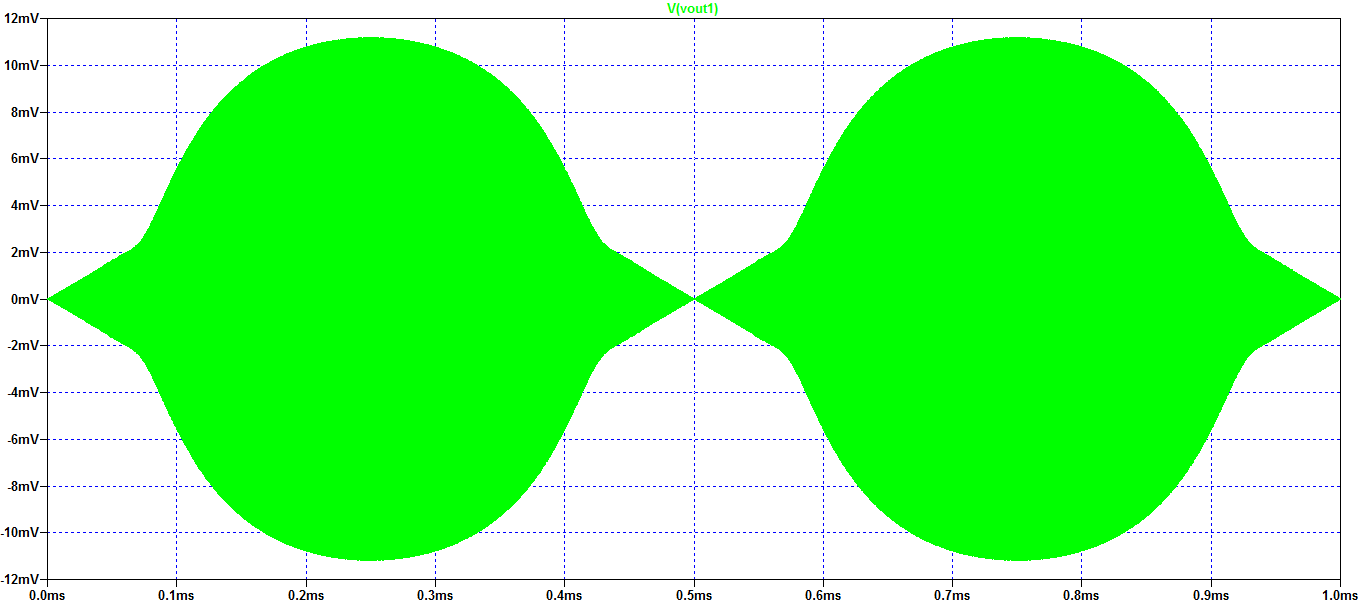Figure 4. Simulation result of VOUT1 for test schematic in Figure 3.

Using the FFT to observe the frequency spectrum of VOUT1 yields the figure below.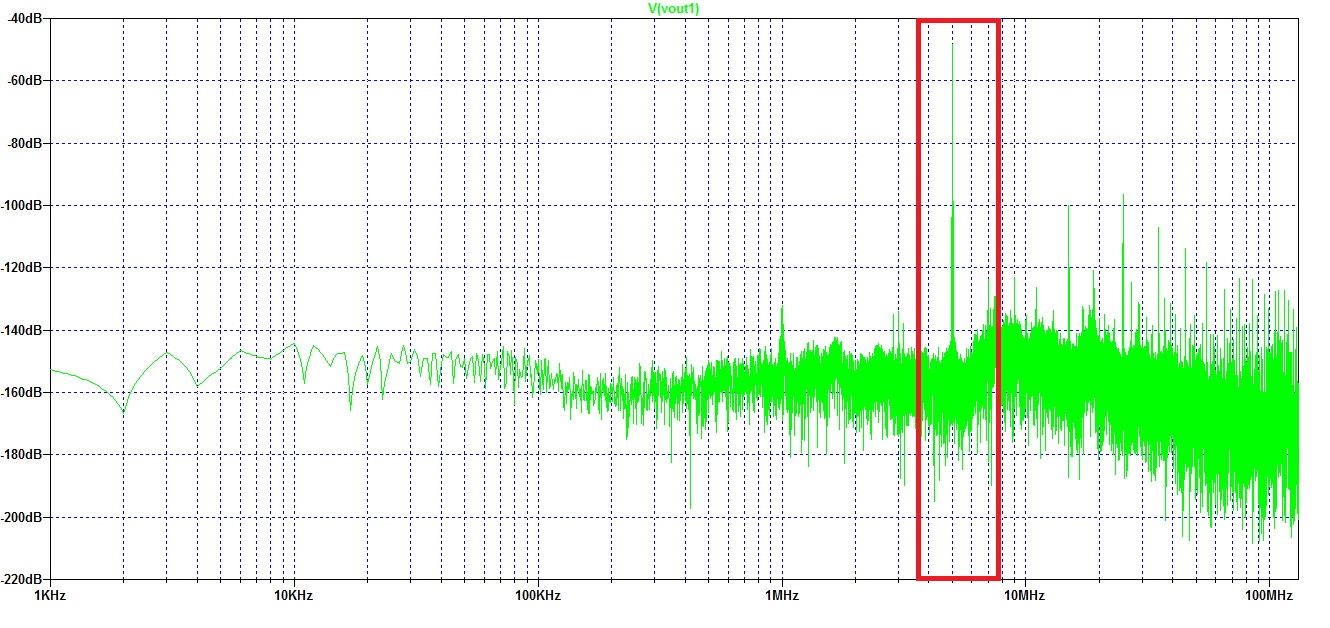Figure 5. FFT of VOUT1Figure 6. Zoom-in view on the peaks of the FFT curve in Figure 5.

From the FFT plot, it is clear that the peaks are at 4.999 MHz and 5.001 MHz, verifying our theoretical expectations. Furthermore, the information in VSIG1 is visible at the envelope of VOUT1. Can you see it?Figure 7. Where is the information of VSIG1?

Now we have an AC signal with information “riding” on it. All we have to do is amplify, then demodulate it before the output. Right? Not yet, there is one more bit of housekeeping that needs meticulous attention, and that is the diodes we choose for the bridge.

What happens when the diodes are mismatched? Let’s find out! Below, I’ve declared a new diode model, V48, with its saturation current at 7pA and zero-bias junction capacitance at 205pF.Figure 8. Modified schematic for the P2 Diode Bridge. D2 has been mismatched.Figure 9. Simulation result of Figure 8.

Mamamia! What has happened to our output? Can you imagine, increasing the saturation current by just 4pA and the capacitance by 100pF having such an effect on the output? To ensure that choice of values wasn’t a factor, I matched all the diodes again using V48, re-simulated, and got the proper waveform. It certainly must have been a daunting task to manufacture the P2 with 4 diodes that had such stringent matching conditions.

This isn’t the only instance matching played a crucial role in design. I believe the same rule applies for Kelvin bridges, or any similar circuit configurations, the only difference being a more relaxed demand in accuracy. How about current mirrors, who have to have MOS pairs that are identical to each other or else the “mirrored” currents would have offsets?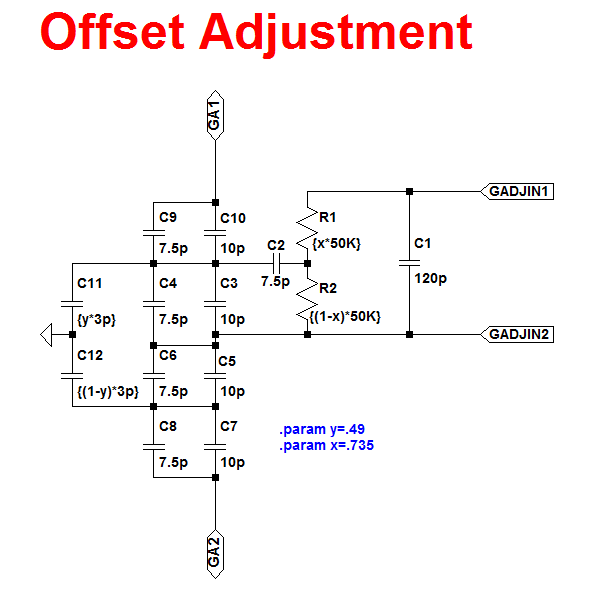Figure 10. Schematic for adjusting offset in the P2.

This block isn’t the only one controlling the gain of the oscillator. Remember the C40 capacitor encircled in red back in part 1? I think it is meant to provide feedback from the AC demodulator (which makes sense, how can you demodulate/decode something if you have no idea or information on the code or original content used?) This discussion however, is for a future article.

Looking closer at the resistor-capacitor network, C11 and C12 are most probably variable capacitors, while R1 and R2 are potentiometers. Terminals GA1 and GA2 connect back to the oscillator while GADJIN1 and GADJIN2 connect to the outputs of the diode bridge. From part 2, we recall the most interesting component of the oscillator, C6, where the value of capacitance and amplitude of oscillation share an inversely proportional relationship, valid from 25pF to 1µF, after which oscillation stops. Looking back at Figure 10, I believe it is safe to assume that the capacitance will only vary in the pF range because series-parallel connections will cancel each other out. We should be surprised if we saw a capacitor here with a value of 1µF or more.

To conclude, let us simulate the schematic in Figure 1.Figure 11. Simulation result of Figure 1.

Node 2 is the output of the diode bridge (shunted to the offset adjustment block). Node 7 is the input pulse to the diode bridge. Different combinations of x and y yield different results (less than 1 of course). When x and y are at very low values, the peak-to-peak voltage of node 2 approaches that of node 7. I think the default values set in Figure 10 are just right to prevent overmodulation.

Can you see the information riding on node 2?